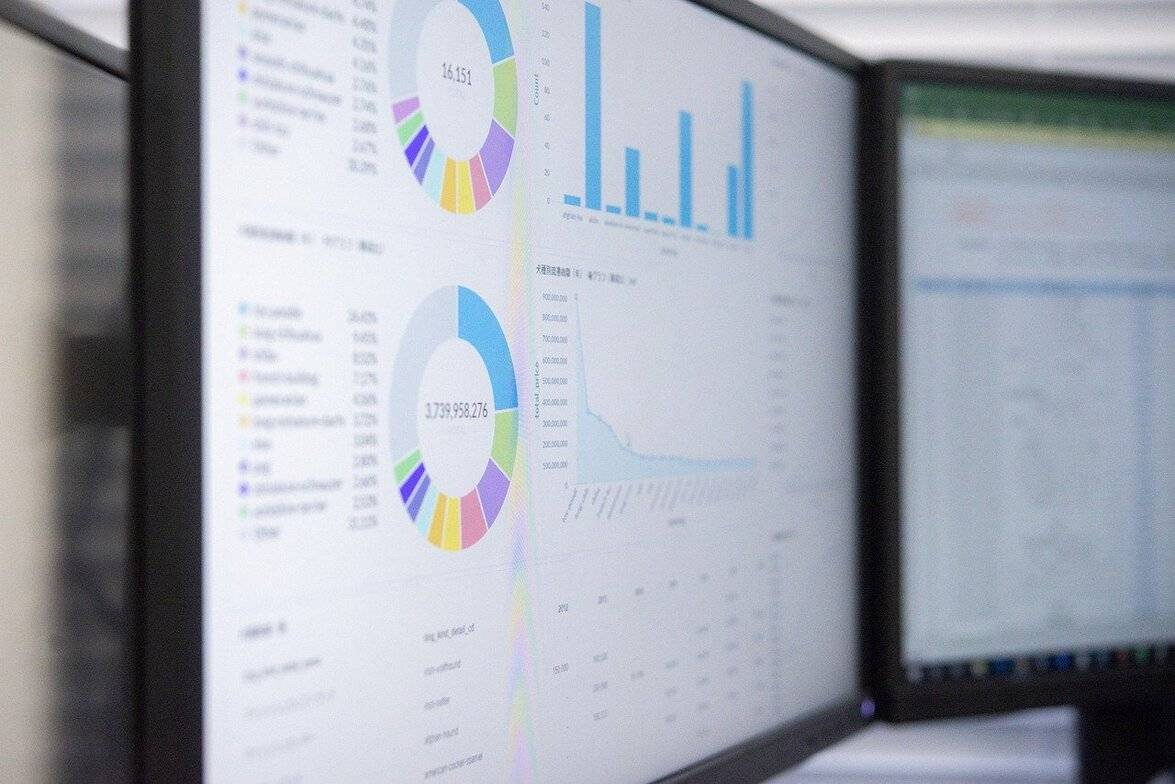# Standardizing the Data of Different Scales - Which Method to Use?

When you're doing data analysis, you might find yourself with a number of different variables to work with. For example, perhaps you have invited participants to your lab and run them through your experiment. You have collected data on variables such as the participant's age (in years), their reaction time to a particular stimulus (in milliseconds), their reaction time to another stimulus (in milliseconds), and their rating of a preference (on a 1 – 10 scale).

When you want to analyse this data to find patterns in it, you first need to make sure that your data variables are compatible with each other. Considering the example above, the two measures of reaction time would be comparable, as they are both measured in milliseconds. But how would you look at, say, the relationship between age and reaction time? To do this, you need to use a statistical technique called standardization.Confusingly, standardization can have several meanings, depending on the field. And to add to the confusion, others may refer to the same process as normalization. The process we are talking about here is defined as the transforming of data of different types to a uniform scale so that they can be compared.

## Methods for standardization

### Standard deviation

The first step in standardization is quantifying how much variance exists in your data. This is described by the standard deviation, which is calculated by working out the square root of the average of the squared deviations of each value from its average value. This will give you a number that represents variance, which you need for the next step.

### Z-score

The most common method for standardization is the calculation of a z-score. To do this, you need to know the mean and standard deviation of the population which your data is drawn from. You calculate a z-score by subtracting the mean of the population from the score in question, and then dividing the difference by the standard deviation of the population. This means that each variable will have a mean of 0 and a standard deviation of 1, so you can compare your different variables meaningfully.

### 0-1 scale

Another option is to take z-scores and adapt them so that they fall on a scale of 0 to 1. Some statistics programs can do this automatically for you. One big advantage of this method is that is lets you eyeball the data very easily, as it's intuitively obvious what the difference between a score of 0.6 and 0.8 is, for example. However, scaling in this manner can sometimes lose data points at the extreme ends, depending on the method used. This is an unnecessary loss of data, so unless there is a strong reason for preferring the 0-1 scale, a z-score is generally sufficient for most purposes.

## Find out more

To fully understand this topic and choose the right method for your data analysis, we recommend reading up on papers by statisticians which cover this issue. Here are some references to get you started:

- Everitt, B.S. Cluster Analysis. 1993. Third Edition. (New York and Toronto: Halsted Press, of John Wiley & Sons Inc.).

- Gower, J. C. 1985. Measures of similarity, dissimilarity, and distance. Pages 397-405 in Encyclopedia of Statistical Sciences, Vol. 5. S. Kotz, N.L. Johnson, and C.B. Read,    Editors. (New York: John Wiley and Sons).

- Johnson, R.A., and D.W. Wichern. 1992. Applied Multivariate Statistical Analysis. 3rd Edition. (Englewood Cliffs, New Jersey: Prentice Hall).

- Milligan, G. W., and M. C. Cooper. 1988. A study of standardization of variables in cluster analysis. Journal of Classification, 5, 181-204.

- van Tongeren, O. F. R. 1995. Cluster Analysis. Pages 174-212 in: R. H. G. Jongman, C. J. F. ter Braak, and O. F. R. van Tongeren, Eds. Data Analysis in Landscape and Community Ecology. (Cambridge & New York: Cambridge University Press).